# Analysis of Variance (ANOVA)

#### ONE-WAY ANOVA

A One-Way Analysis of Variance is a way to test the equality of three or more sample means, all of which are independent, of a single variable. Although the 2-sample t-Test is similar but iin order to compare k samples, you will need to run [k*(k-1)/2] number of tests. It is called ANOVA because it compares group means by analyzing comparisons of variance estimates. Total variation is split into variation between subgroups and variation within subgroups. If variation between subgroups is much larger than the variation within subgroups, it is likely that the sample means are different.

##### Hypotheses

The null hypothesis is, all population means are equal and the alternative hypothesis is, at lleast one mean is different.

##### Assumptions

In order for the test to be reliable, the following conditions must be met:

1. Samples are independently and randomly drawn from the source populations.
2. The variances of the populations must be equal.
3. Samples must be random.
4. Residuals are random and normally distributed with approximately zero mean.
5. Note: the samples are all the same size, the test is relatively robust for data which are not normally distributed and have unequal variances.

##### Model

Xij =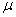+ ai + +eij
whereis the overall mean
ai is the effect of factor A of level i
eij is the error term

##### Variation components

Total Sum of Squares (Total Variance):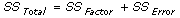Factor Sum of Squares (Variation between subgroups):Error Sum of Squares (Variation within subgroups):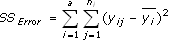Mean Sum of Squares (Total):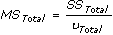Mean Sum of Squares (Factor):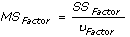Mean Sum of Squares (Error):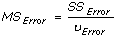Test Statistic is based on F-distribution: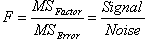Reject H0 if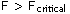#### TWO-WAY ANOVA

The Two-Way Analysis of Variance is an extension to the One-Way Analysis of Variance. It is a way of studying the effects of two factors separately (main effects) and sometimes together (interaction effect). In One-Way ANOVA we can test k levels of 1 factor and in Two-Way ANOVA we have k*q levels of 2 factors. The two independent variables are called factors and they affect the dependent variable. Each factor will have two or more levels within it. In Two-Way ANOVA, there are two main types of effects, main and interaction effects. The main effect is the effects on the dependent variable due to the independent variables one at a time. This is similar to the One-Way ANOVA. The interaction effect is the effect that one factor has on the other factor.

##### Hypotheses

There are three sets of hypothesis with the two-way ANOVA. The means of different levels of the first factor are equal. The means of different levels of the second factor are equal. There is no interaction between the two factors.

##### Assumptions

In order for the test to be reliable, the following conditions must be met:

1. The populations from which the samples were obtained must be normally or approximately normally distributed.
2. The samples must be independent.
3. The variances of the populations must be equal.
4. The groups must have the same sample size.

##### Model

Xijk =+ ai + bj + (ab)ij +eijk
whereis the overall mean
Ai is the effect of factor A of level i
Bj is the effect of factor B of level j
(AB)ij is the interaction effect of level i and j
eijk is the error term